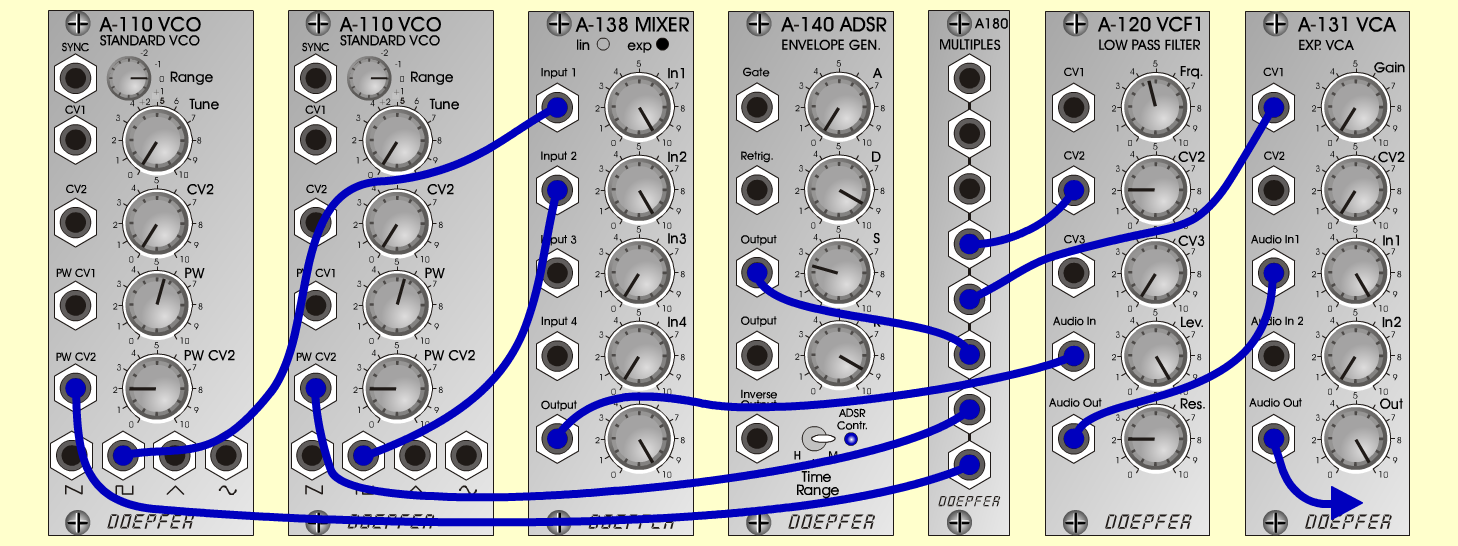SF-WurlitzerKeyboardsD o e p f e r A - 100 Connections: Settings: A-110/1 (Pulse)  <=>  A-138 (Input 1)   A-110/1 (Pulse)  <=>  A-138 (Input 2)   A-140 (Output)  <=>  A-180   A-180 <=>  A-110/1 (PW CV 2)   A-180 <=>  A-110/2 (PW CV 2)   A-180 <=>  A-120 (CV 2)   A-180 <=>  A-131 (CV 1)   A-138 (Output)  <=>  A-120 (Audio In)   A-120 (Audio Out)  <=>  A-131 (Audio In)   A-131 (Audio Out)  <=> Amplifier   Pitch, Trigger and Gate: KB A-110/1 (PW = 5.5, PW CV 2 = 2)   A-110/2 (PW = 5.5, PW CV 2 = 2)   A-138 (In 1 = 10, In 2 = 10, Out = 10)   A-120 (Freq = 4.5, CV 2 = 2, Lev = 10, Res = 2)   A-140 (A = 0, D = 9, S = 2.5, R = 9, Range = M)   A-131 (Gain = 0, Audio In 1 = 10,  Audio Out = 10) Notes: Tune "VCO 2"  4 Cents higher than "VCO 1".   (From german magazin KEYBOARDS,   Feb 2001, page 76, Soundforum) Josef MuellerSound sample SF-Wurlitzer